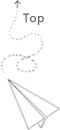## 數據類型

GLSL 有C語言及其他語言大多都有的 type:int, float, double, uint, bool，另位還有兩種容器 type: 向量(Vector)跟矩陣(Matrix)

• 向量

• 可以有 $[1, 4]$ 個分量
• vecn 包含 $n$ 個 float
• bvecn 包含 $n$ 個 bool
• ivecn 包含 $n$ 個 int
• uvecn 包含 $n$ 個 unsigned int
• dvecn 包含 $n$ 個 double
• 分量用 .x, .y, .z, .w 來存取分量，或是rgba(顏色)跟stpq(材質)來存取。

• 重組(Swizzling)

• 可以用分量的組合來建立新的 vec

## 輸入與輸出

GLSL 可以用 in, out 來標示一個變數是傳入還是傳出，上個 Shader 所指定的 out 會對應到下個 Shader 所指定的 in 變數，名稱要相同。

layout (location = 0) 可以指定 Vertex Attribute 的 Index 這在傳入 Vertex Data 之後，可以用 glVertexAttribPointer() 來描述頂點資料是怎麼排列的，讓 OpenGL 可以正確的繪製## Uniform

Uniform 是 CPU 向 GPU 的 Shader 發送數據的方式，但跟 Vertex Attribute 有點不同。
Uniform 可以在所有的 Shader 中存取，而不用像 Vertex Attribute 是要用 in, out 傳資料，意思是 Uniform 是 Global 的變數，它必須是 獨特的(Unique) 的。

• 如果宣告了一個 uniform 卻沒有使用，編譯器可能會移除該變數，導致在最後編譯出的版本不會包含它

• 傳入 uniform

• 使用 glGetUniformLocation() 以及 glUniform4f()

f float
i int
ui unsigned int
3f 三個 float
fv array of float## 多個頂點屬性## 推薦文章